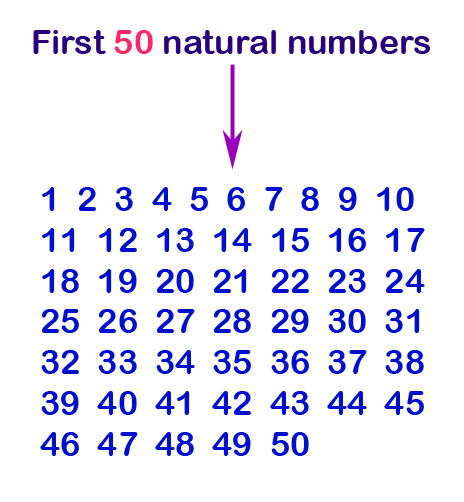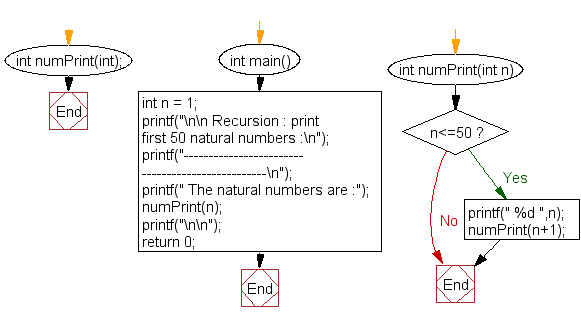﻿ C Program: Print first 50 natural numbers - w3resource

# C Exercises: Print first 50 natural numbers

## C Recursion : Exercise-1 with Solution

Write a program in C to print the first 50 natural numbers using recursion.

Pictorial Presentation:Sample Solution:

C Code:

``````#include<stdio.h>
int  numPrint(int);
int main()
{
int n = 1;
printf("\n\n Recursion : print first 50 natural numbers :\n");
printf("-------------------------------------------------\n");
printf(" The natural numbers are :");
numPrint(n);
printf("\n\n");
return 0;
}
int numPrint(int n)
{
if(n<=50)
{
printf(" %d ",n);
numPrint(n+1);
}
}
```
```

Sample Output:

```Recursion : print first 50 natural numbers :
-------------------------------------------------
The natural numbers are : 1  2  3  4  5  6  7  8  9  10  11  12  13  14  15  16  17  18  19  20  21  22  23
24  25  26  27  28  29  30  31  32  33  34  35  36  37  38  39  40  41  42  43  44  45  46  47  48  49  50
```

Explanation:

```int numPrint(int n)
{
if(n<=50)
{
printf(" %d ",n);
numPrint(n+1);
}
}
```

The above function numPrint() takes an integer n as input and prints the numbers from n to 50 recursively. The base case is when n becomes greater than 50, the function stops executing.

Time complexity and space complexity:

The time complexity of the numPrint() function is O(50-n), since the function will recursively call itself up to 50 times.

The space complexity of this function is O(1), since it does not use any additional memory that depends on the size of the input.

Flowchart:C Programming Code Editor:

Have another way to solve this solution? Contribute your code (and comments) through Disqus.

What is the difficulty level of this exercise?

Test your Programming skills with w3resource's quiz.

﻿

## C Programming: Tips of the Day

__FILE__ macro shows full path

```#include <string.h>

#define __FILENAME__ (strrchr(__FILE__, '/') ? strrchr(__FILE__, '/') + 1 : __FILE__)
For Windows use '\\' instead of '/'.
```

Ref : https://bit.ly/3iEWRoT

We are closing our Disqus commenting system for some maintenanace issues. You may write to us at reach[at]yahoo[dot]com or visit us at Facebook